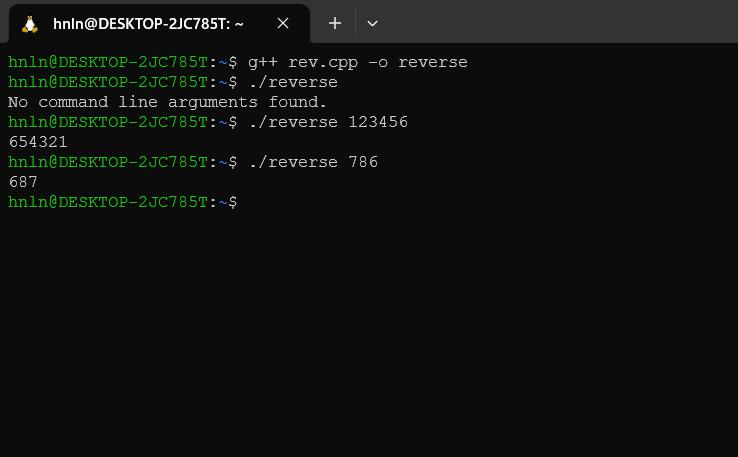Open In App

# TCS Coding Practice Question | Reverse a Number

Given a number, the task is to reverse this number using Command Line Arguments. Examples:

`Input: num = 12345Output: 54321Input: num = 786Output: 687`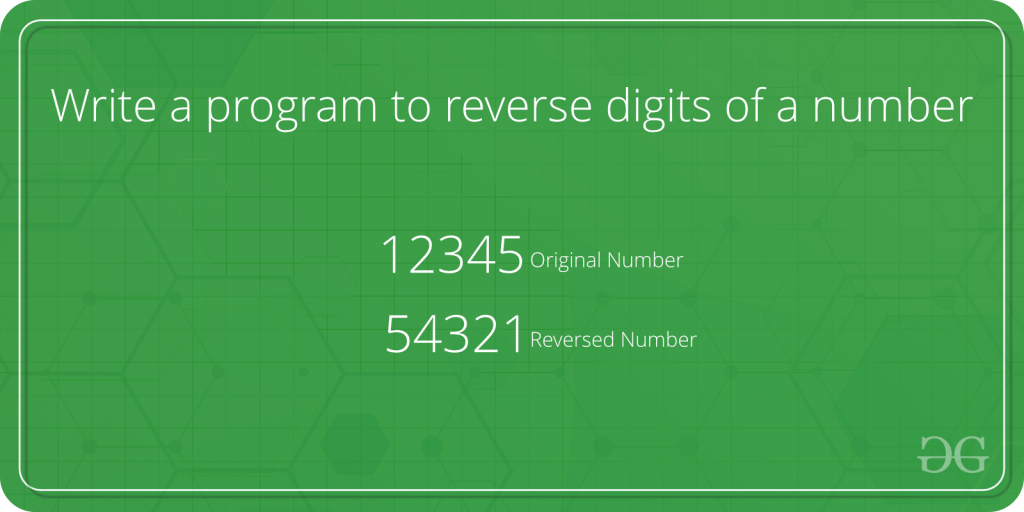Approach:

• Since the number is entered as Command line Argument, there is no need for a dedicated input line
• Extract the input number from the command line argument
• This extracted number will be in String type.
• Convert this number into integer type and store it in a variable, say num
• Initialize a variable, say rev_num, to store the reverse of this number with 0
• Now loop through the number num till it becomes 0, i.e. (num > 0)
• In each iteration,
• Multiply rev_num by 10 and add remainder of num to it. This will store the last digit of num in rev_num
• Divide num by 10 to remove the last digit from it.
• After the loop has ended, rev_num has the reverse of num.

Program:

## C++

 `// C++ program to reverse a number``// using command line arguments` `#include ``#include ``#include ``using` `namespace` `std;` `// Function to reverse the number``int` `reverseNumber(``int` `num)``{` `    ``// Variable to store the``    ``// resultant reverse number``    ``int` `rev_num = 0;` `    ``// Traverse through the number digit by digit``    ``while` `(num > 0) {` `        ``// Append the last digit of num``        ``// as the next digit of rev_num``        ``rev_num = rev_num * 10 + num % 10;` `        ``// Remove the last digit from the num``        ``num = num / 10;``    ``}` `    ``// Return the reversed number``    ``return` `rev_num;``}` `// Driver code``int` `main(``int` `argc, ``char``* argv[])``{` `    ``int` `num;` `    ``// Check if the length of args array is 1``    ``if` `(argc == 1)``        ``cout << ``"No command line arguments found.\n"``;``    ``else` `{` `        ``// Get the command line argument and``        ``// Convert it from string type to integer type``        ``// using function "atoi( argument)"``        ``num = ``atoi``(argv);` `        ``// Reverse the number and print it``        ``cout << reverseNumber(num) << endl;``    ``}``    ``return` `0;``}`

## C

 `// C program to reverse a number``// using command line arguments` `#include ``#include      /* atoi */` `// Function to reverse the number``int` `reverseNumber(``int` `num)``{` `    ``// Variable to store the``    ``// resultant reverse number``    ``int` `rev_num = 0;` `    ``// Traverse through the number digit by digit``    ``while` `(num > 0) {` `        ``// Append the last digit of num``        ``// as the next digit of rev_num``        ``rev_num = rev_num * 10 + num % 10;` `        ``// Remove the last digit from the num``        ``num = num / 10;``    ``}` `    ``// Return the reversed number``    ``return` `rev_num;``}` `// Driver code``int` `main(``int` `argc, ``char``* argv[])``{` `    ``int` `num;` `    ``// Check if the length of args array is 1``    ``if` `(argc == 1)``        ``printf``(``"No command line arguments found.\n"``);``    ``else` `{` `        ``// Get the command line argument and``        ``// Convert it from string type to integer type``        ``// using function "atoi( argument)"``        ``num = ``atoi``(argv);` `        ``// Reverse the number and print it``        ``printf``(``"%d\n"``, reverseNumber(num));``    ``}``    ``return` `0;``}`

## Java

 `// Java program to reverse a number``// using command line arguments` `class` `GFG {` `    ``// Function to reverse the number``    ``public` `static` `int` `reverseNumber(``int` `num)``    ``{` `        ``// Variable to store the``        ``// resultant reverse number``        ``int` `rev_num = ``0``;` `        ``// Traverse through the number digit by digit``        ``while` `(num > ``0``) {` `            ``// Append the last digit of num``            ``// as the next digit of rev_num``            ``rev_num = rev_num * ``10` `+ num % ``10``;` `            ``// Remove the last digit from the num``            ``num = num / ``10``;``        ``}` `        ``// Return the reversed number``        ``return` `rev_num;``    ``}` `    ``// Driver code``    ``public` `static` `void` `main(String[] args)``    ``{` `        ``// Check if length of args array is``        ``// greater than 0``        ``if` `(args.length > ``0``) {` `            ``// Get the command line argument and``            ``// Convert it from string type to integer type``            ``int` `num = Integer.parseInt(args[``0``]);` `            ``// Reverse the number and print it``            ``System.out.println(reverseNumber(num));``        ``}``        ``else``            ``System.out.println(``"No command line "``                               ``+ ``"arguments found."``);``    ``}``}`

## Python

 `import` `sys` `# Function to reverse the number``def` `reverseNumber(num):``    ``# Variable to store the resultant reverse number``    ``rev_num ``=` `0` `    ``# Traverse through the number digit by digit``    ``while` `num > ``0``:``        ``# Append the last digit of num as the next digit of rev_num``        ``rev_num ``=` `rev_num ``*` `10` `+` `num ``%` `10` `        ``# Remove the last digit from the num``        ``num ``=` `num ``/``/` `10` `    ``# Return the reversed number``    ``return` `rev_num` `# Driver code``if` `__name__ ``=``=` `"__main__"``:``    ``if` `len``(sys.argv) ``=``=` `1``:``        ``print``(``"No command line arguments found."``)``    ``else``:``        ``# Get the command line argument and convert it from string to integer``        ``num ``=` `int``(sys.argv[``1``])` `        ``# Reverse the number and print it``        ``print``(reverseNumber(num))`

Output:

• In C: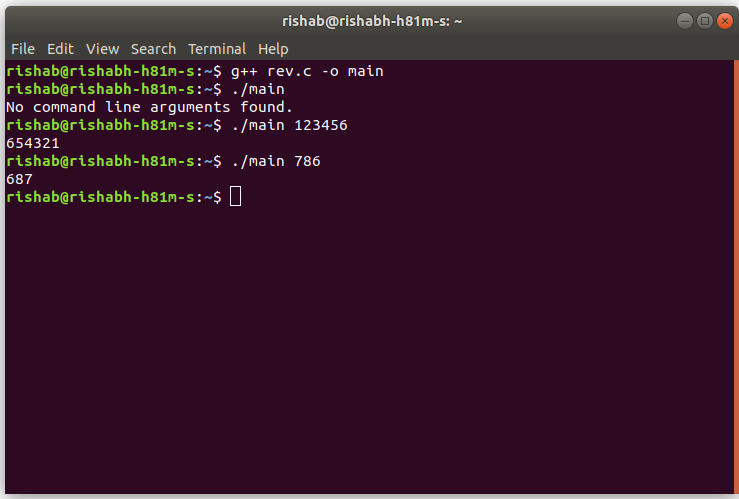• In Java :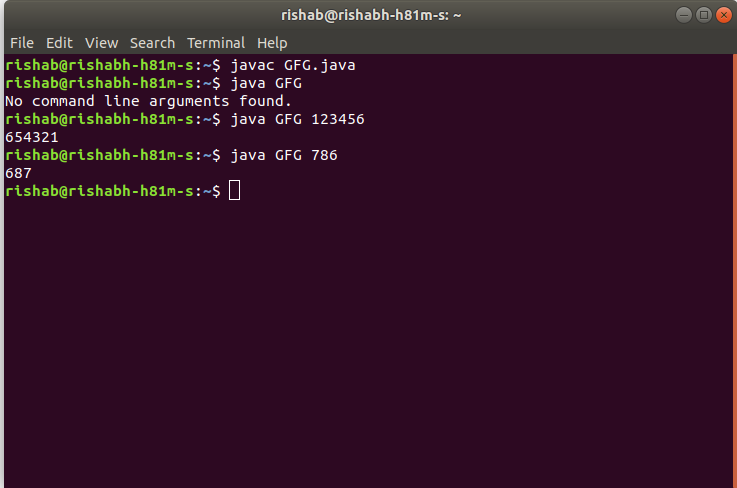• In Python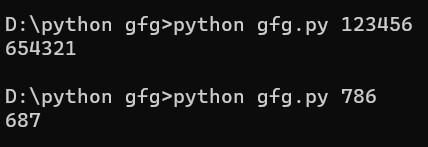• In C++: